Functional programming’s been quite the eye-opening journey for me. This post, and posts like it, are an attempt to share my insights and perspectives as I trek new functional programming lands.

Ramda’s been my go-to FP library because of how much easier it makes functional programming in JavaScript. I highly recommend it.

### Pipe

The concept of `pipe` is simple — it combines `n` functions. It’s a pipe flowing left-to-right, calling each function with the output of the last one.

Let’s write a function that returns someone’s `name`.

``````getName = (person) => person.name;

``````

Let’s write a function that uppercases strings.

``````uppercase = (string) => string.toUpperCase();

``````

So if we wanted to get and capitalize `person`'s name, we could do this:

``````name = getName({ name: 'Buckethead' });
uppercase(name);

``````

That’s fine but let’s eliminate that intermediate variable `name`.

``````uppercase(getName({ name: 'Buckethead' }));
``````

Better, but I’m not fond of that nesting. It can get too crowded. What if we want to add a function that gets the first 6 characters of a string?

``````get6Characters = (string) => string.substring(0, 6);

// 'Bucket'
``````

Resulting in:

``````get6Characters(uppercase(getName({ name: 'Buckethead' })));

// 'BUCKET';
``````

Let’s get really crazy and add a function to reverse strings.

``````reverse = (string) =>
string
.split('')
.reverse()
.join('');

// 'daehtekcuB'
``````

Now we have:

``````reverse(get6Characters(uppercase(getName({ name: 'Buckethead' }))));
// 'TEKCUB'
``````

It can get a bit…much.

### Pipe to the rescue!

Instead of jamming functions within functions or creating a bunch of intermediate variables, let’s `pipe` all the things!

``````pipe(
getName,
uppercase,
get6Characters,
reverse
// 'TEKCUB'
``````

Pure art. It’s like a todo list!

Let’s step through it.

For demo purposes, I’ll use a `pipe` implementation from one of Eric Elliott’s functional programming articles.

``````pipe = (...fns) => (x) => fns.reduce((v, f) => f(v), x);
``````

I love this little one-liner.

Using rest parameters, see my article on that, we can pipe `n` functions. Each function takes the output of the previous one and it’s all reduced ? to a single value.

And you can use it just like we did above.

``````pipe(
getName,
uppercase,
get6Characters,
reverse
// 'TEKCUB'
``````

I’ll expand `pipe` and add some debugger statements, and we’ll go line by line.

``````pipe = (...functions) => (value) => {
debugger;

return functions.reduce((currentValue, currentFunction) => {
debugger;

return currentFunction(currentValue);
}, value);
};
``````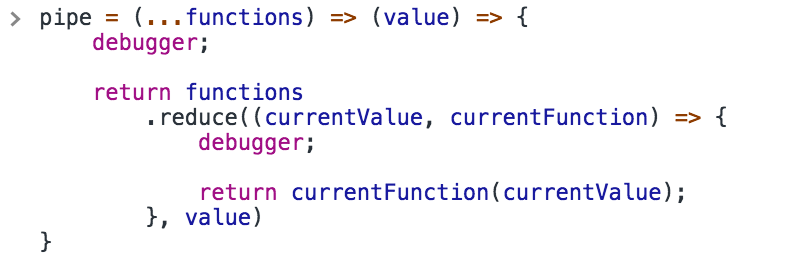Call `pipe` with our example and let the wonders unfold.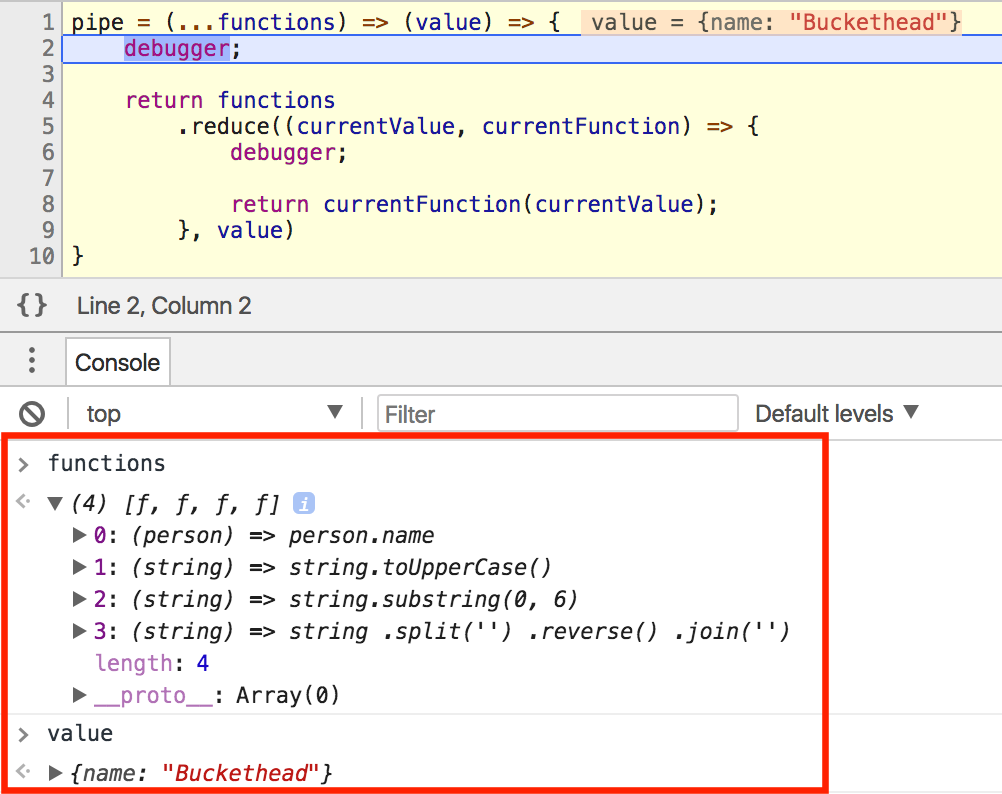Check out the local variables. `functions` is an array of the 4 functions, and `value` is `{ name: 'Buckethead' }`.

Since we used rest parameters, `pipe` allows any number of functions to be used. It’ll just loop and call each one.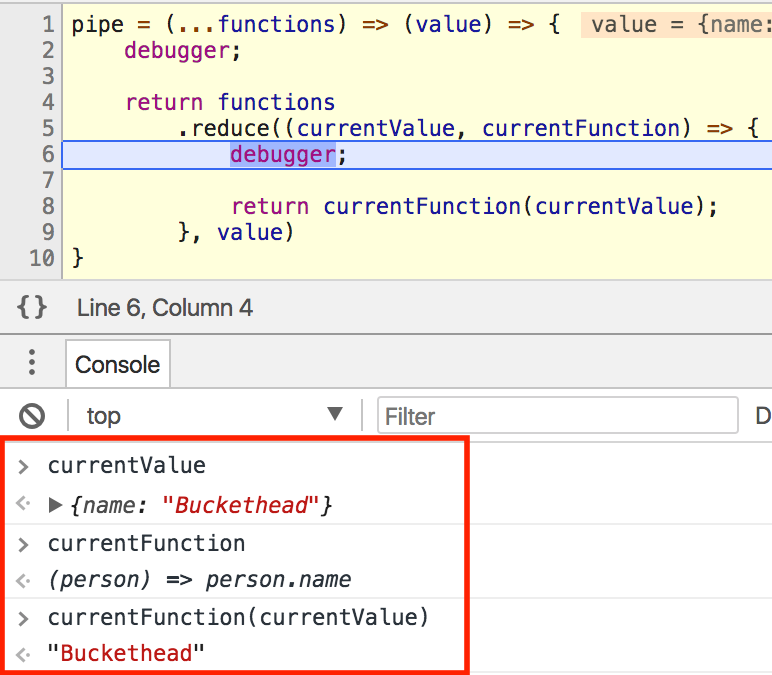On the next debugger, we’re inside `reduce`. This is where `currentValue` is passed to `currentFunction` and returned.

We see the result is `'Buckethead'` because `currentFunction` returns the `.name` property of any object. That will be returned in `reduce`, meaning it becomes the new `currentValue` next time. Let’s hit the next debugger and see.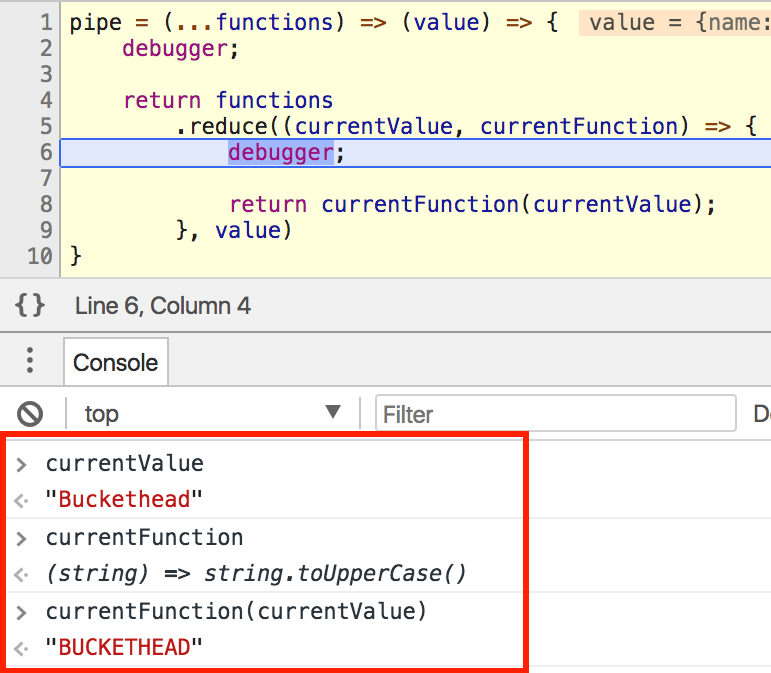Now `currentValue` is `‘Buckethead’` because that’s what got returned last time. `currentFunction` is `uppercase`, so `'BUCKETHEAD'` will be the next `currentValue`.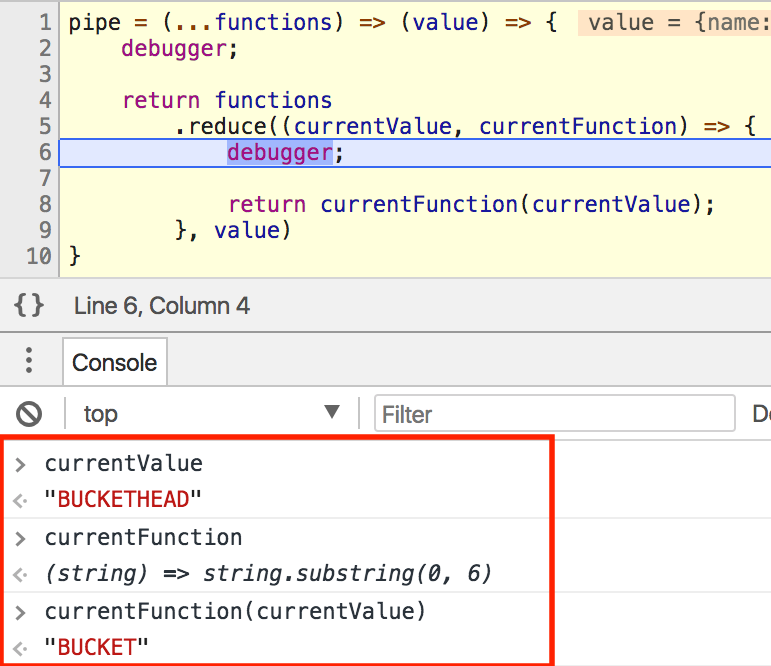The same idea, pluck `‘BUCKETHEAD’`'s first 6 characters and hand them off to the next function.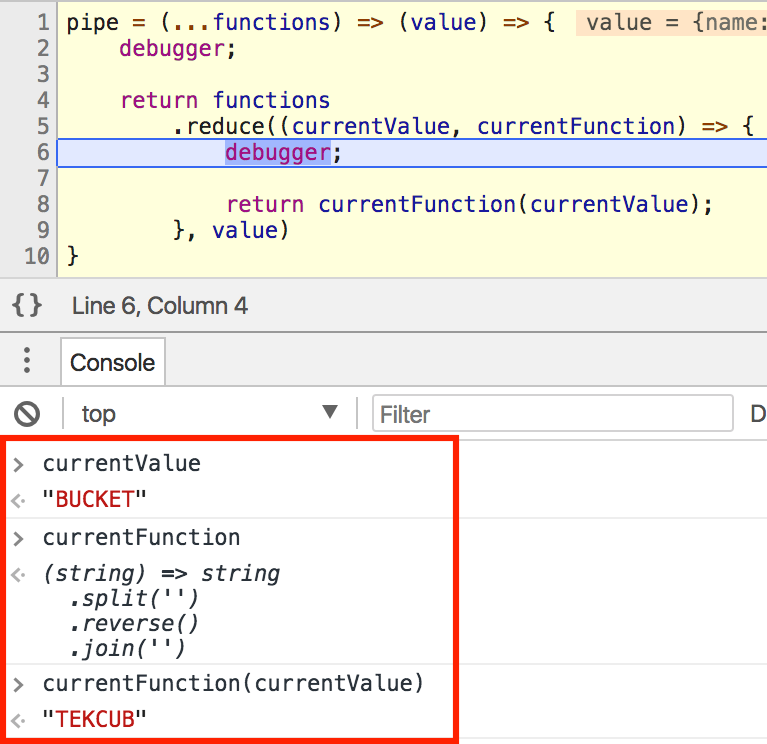`reverse(‘.aedi emaS’)`And you’re done!

It’s just `pipe` in the other direction.

So if you wanted the same result as our `pipe` above, you’d do the opposite.

``````compose(
reverse,
get6Characters,
uppercase,
getName
Notice how `getName` is last in the chain and `reverse` is first?
Here’s a quick implementation of `compose`, again courtesy of the Magical Eric Elliott, from the same article.
``````compose = (...fns) => (x) => fns.reduceRight((v, f) => f(v), x);
I’ll leave expanding this function with `debugger`s as an exercise to you. Play around with it, use it, appreciate it. And most importantly, have fun!